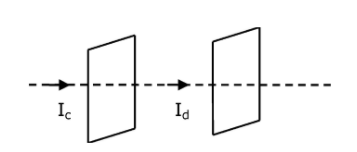Checkout JEE MAINS 2022 Question Paper Analysis : Checkout JEE MAINS 2022 Question Paper Analysis :

# Seawater at a frequency f = 9 × 102 Hz, has permittivity ∈ = 80∈0 and resistivity ρ = 0.25 Ωm.

Seawater at a frequency f = 9 × 102 Hz, has permittivity ∈ = 80∈0 and resistivity ρ = 0.25 Ωm. Imagine a parallel plate capacitor is immersed in seawater and is driven by an alternating voltage source V(t) = V0 sin(2πft). Then the conduction current density becomes 10x times the displacement current density after time t= 1800. The value of x is __________.

(Given : 1/4𝜋∈0 = 9 × 109Nm2C-2)Given:-

f = 9 × 102 Hz

∈=∈0r

∈=80∈0

So, ∈r=80

ρ = 0.25 Ωm

V(t) = V0 sin (2πft)

Displacement current, Id = dq/dt=(cdv/dt)

$$\begin{array}{l}I_{d}=\frac{\epsilon _{0}\epsilon _{r}A}{d}\frac{d}{dt}(v_{0}sin(2\pi ft))\end{array}$$

$$\begin{array}{l}I_{d}=\frac{\epsilon _{0}\epsilon _{r}A}{d}V_{0}(2\pi f)cos(2\pi ft)—–(1)\end{array}$$

Where d is the distance between plates & conduction current Ic = V/R

$$\begin{array}{l}I_{c}= \frac{V_{0}sin(2\pi ft)}{\rho \frac{d}{A}}=\frac{AV_{0}sin(2\pi ft)}{\rho d}——(2)\end{array}$$

Divide equation (1) and (2)

$$\begin{array}{l}\frac{I_{d}}{I_{c}}= \epsilon _{0}\epsilon _{r}2\pi f(\rho )cot(2\pi ft)\end{array}$$

$$\begin{array}{l}\frac{I_{d}}{I_{c}}= \frac{1}{4\pi \times 9\times 10^{9}}\times 80\times 2\pi \times 9\times 10^{2}\times (0.25)\times cot(2\pi \times9\times 10^{2}\times \frac{1}{800} )\end{array}$$

$$\begin{array}{l}= \frac{10^{3}}{10^{9}}(cot(\frac{9\pi }{4}))\end{array}$$

$$\begin{array}{l}= \frac{10^{3}}{10^{9}}\end{array}$$

$$\begin{array}{l}\frac{I_{d}}{I_{c}}=\frac{1}{10^{6}}\end{array}$$

Ic = 106 Id

So x = 6(6)(3)